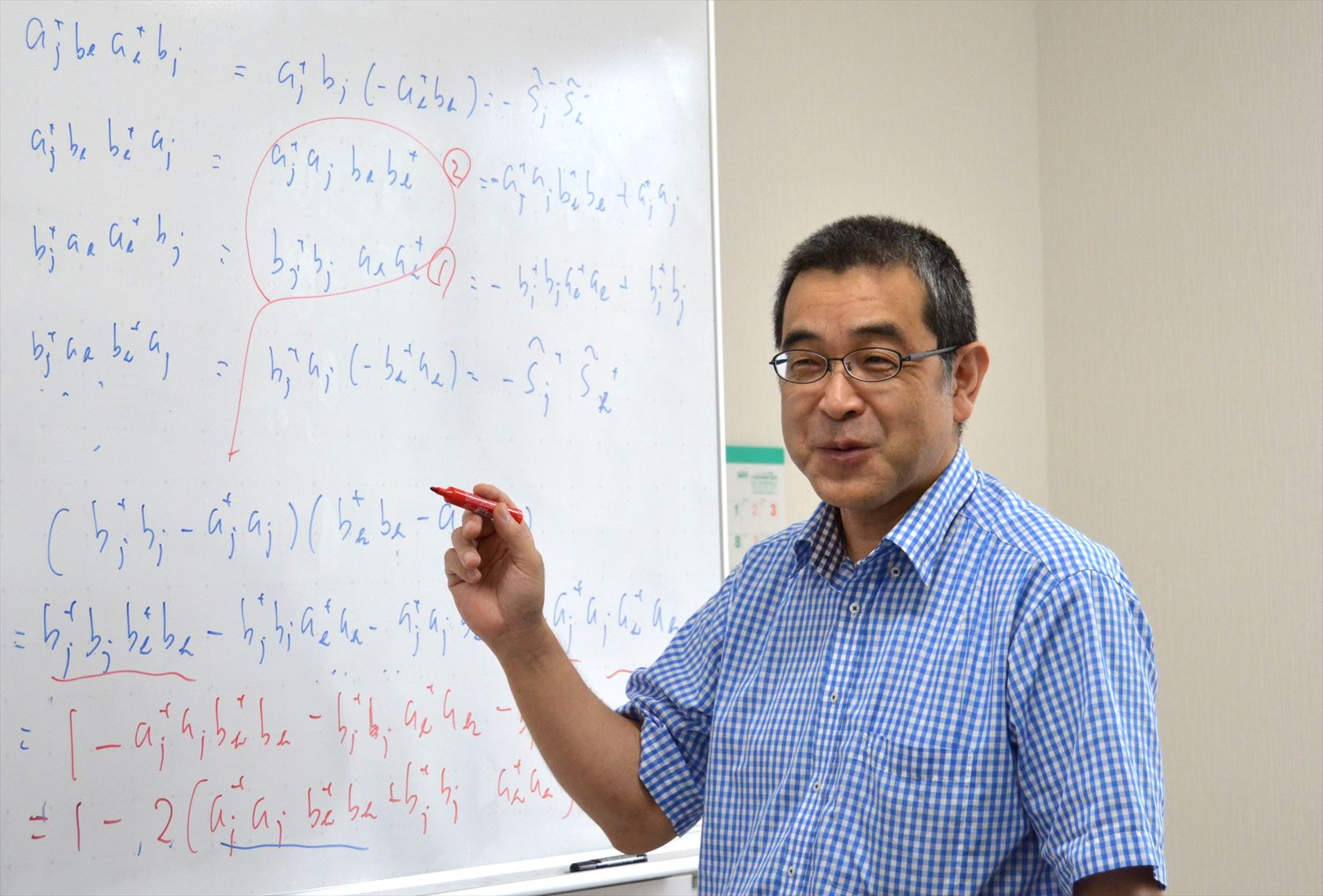### The principle Feynman and Einstein could not find was discovered ~Why Faraday’s law and Lorentz force create the same electromotive force?~

Tsukuba, Japan – The Faraday’s induction formula (flux rule) of electromagnetism says that the electromotive force (emf) created in a conducting circuit is equal to the rate at which the magnetic flux through the conducting circuit changes as it is written on a high school text in physics. This emf can be calculated in two ways: either by using the Lorentz force formula and calculating the force acting on electrons in the moving conductor of the circuit; or via one of Maxwell’s equations (Faraday’s law) and calculating the change of the magnetic flux penetrating through the circuit. The Lorentz force formula and Maxwell’s equations are two distinct physical laws, yet the two methods yield the same results.

Why the two results coincide was not known. In other words, the flux rule consists of two physically different laws in classical theories. Interestingly, this problem was also a motivation behind the development of the theory of relativity by Albert Einstein. In 1905, Einstein wrote in the opening paragraph of his first paper on relativity theory, “It is known that Maxwell’s electrodynamics – as usually understood at the present time – when applied to moving bodies, leads to asymmetries which do not appear to be inherent in the phenomena.” But Einstein’s argument moved away from this problem and formulated special theory of relativity, thus the problem was not solved.
Richard Feynman once described this situation in his famous lecture (The Feynman Lectures on Physics, Vol. II, 1964), “we know of no other place in physics where such a simple and accurate general principle requires for its real understanding an analysis in terms of two different phenomena. Usually such a beautiful generalization is found to stem from a single deep underlying principle. ･･････ We have to understand the “rule” as the combined effects of two quite separate phenomena.”Dr. Hiroyasu Koizumi’s study, recently published in the Journal of Superconductivity and Novel Magnetism, has revealed what is the “single deep underlying principle” in the “flux rule” envisaged by Feynman. It is a duality of the U(1) phase factor added on the wave function; it describes the translational motion of electrons, and also gives a time-dependent gauge potential that induces an effective electric field on the electrons. The former view corresponds to the result obtained by the Lorentz force formula, and the latter to the result using the Maxwell’s equation for the Faraday’s law.

#### Read full text ->   University of Tsukuba

Article: “Flux Rule, U (1) Instanton, and Superconductivity”, Journal of Superconductivity and Novel Magnetism at DOI: 10.1007/s10948-017-4302-3.